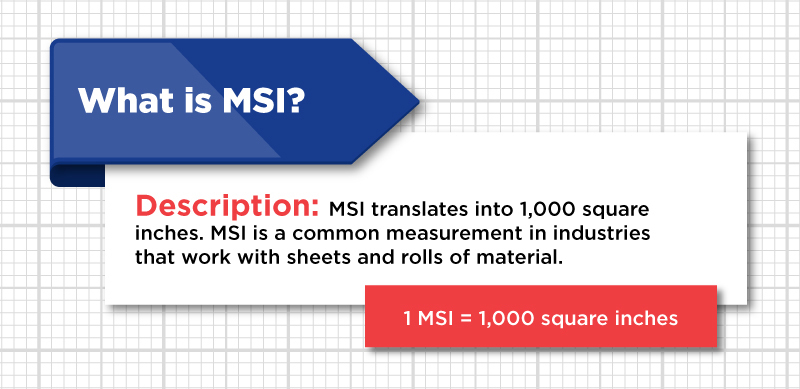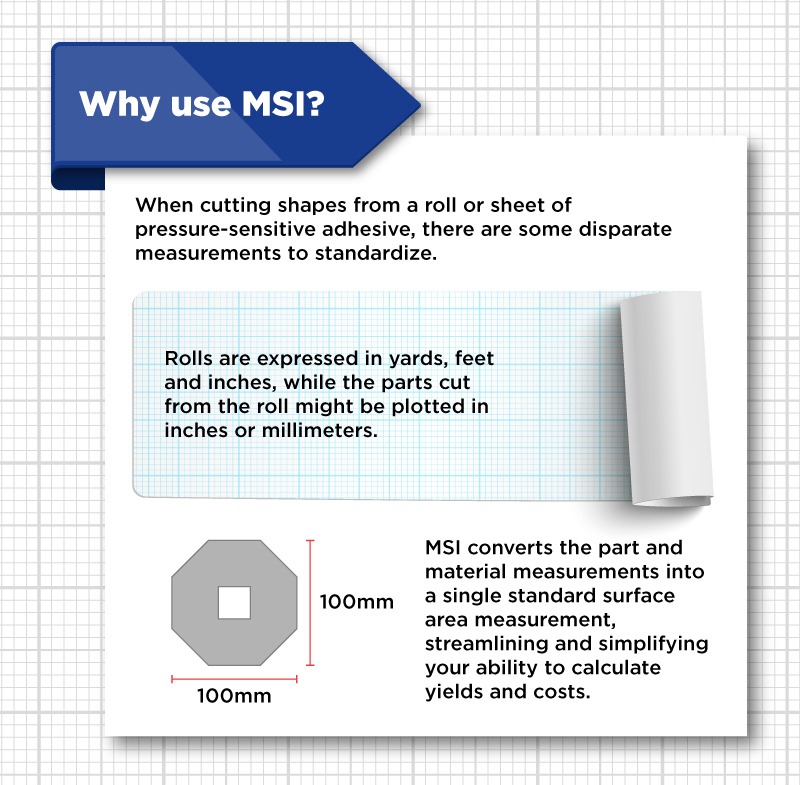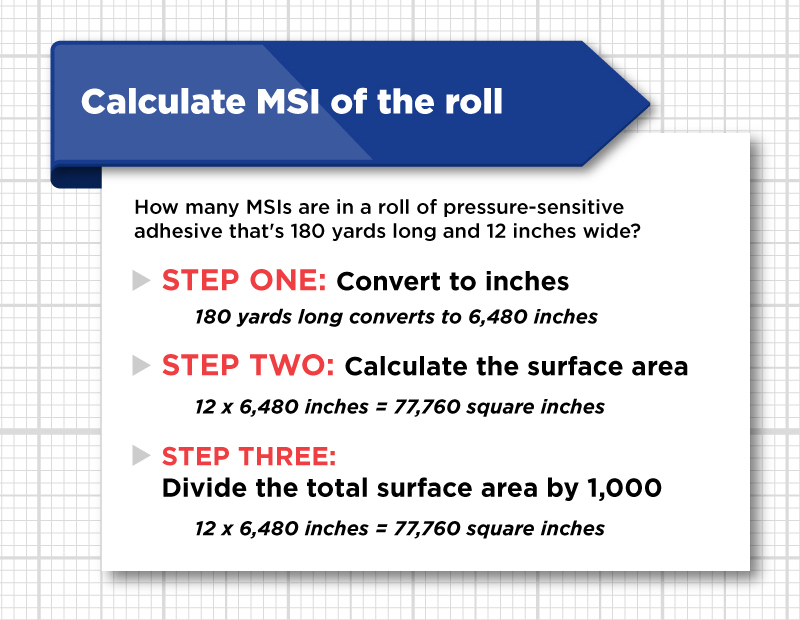Categories

# measurements?

MSI is a unit of measurement per roll (or piece) that is common in the U.S. printing industry. Literally, it equates to “a thousand square inches.” (Another common unit is MSF, which is 1,000 square feet.)

• 1 MSI = 1,000 square inches### Why is MSI measurement needed?

MSI offers a streamlined way to convey the area of a roll or sheet you plan to convert to an adhesive coated material. When choosing materials, the quoted price would ultimately be expressed in cost per MSI.

A natural starting point is calculating the MSI of the roll of your chosen material.## What can you do with an MSI measurement?

Once you have MSI per roll/sheet you can:

• Convert and compare different sheet/roll sizes
• Find percentage of usable material on a piece or roll
• Calculate and compare prices

Calculating the MSI of your project can be easily accomplished on a spreadsheet or by using this online calculator tool.

Once you’ve calculated the MSI of the material, you can find the price per MSI.

## How to use the MSI calculator

What you’ll need is the raw measurements of your roll

• Width in inches
• Length in feet
• The number of rolls and pieces that will be used in the project. (If this is unknown, start by entering a value of 1.)

Before entering height and width into the calculator:

• Convert the fractions into decimals.
• Do not include units.

Here’s an example:

• Entering 5.5 in the roll width field is correct.
• Entering 5.5”, 5.5 inches or 5.5 in. are incorrect## How to calculate MSI per roll measurement

The following will break down, step by step, with examples, how to make an MSI calculation for your roll or sheet of material. Let’s say you have one roll that is 2,000 feet long and 5 ½ inches wide. To calculate the MSI, you’ll want to complete the following steps:

• Convert the fractions to decimals
(5 ½ inch width equals 5.5 inches)
• Convert feet to inches
(2,000 feet equals 24,000 inches)
• Find the total area by multiplying length times height
(5.5 times 24,000 equals 132,000)
• Finally, to get the MSI per roll measurement, divide total surface area by 1,000
(132,000 divided by 1,000 equals 132 MSI per roll)

MSI is a relatively simple calculation, once you wrap your mind around it. But using the online calculator reduces the opportunity for human error and streamlines the process.

Fill in the required dimensions and click on "Calculate" to figure the "Total MSI`s". Do not type units, or fractions into the entry boxes. For example, enter 5.5 for inches, not 5.5" , 5.5 inches, or 5.5 in.

 Number of Rolls / Pieces * Roll / Piece Width * (Inches) Roll / Piece Length * (Feet) Total MSI's Ready to make your plan a reality?